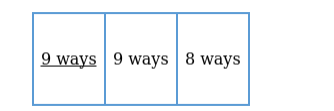# How many 3-digit numbers are there with no digit repeated?

Question:

How many 3-digit numbers are there with no digit repeated?

Solution:

Given: We have 10 numbers i.e. 0,1,2,3,4,5,6,7,8,9

To Find: Number of 3-digit numbers formed with no repetition of digits.

Conditions: No digit is repeated

Let us represent the 3-digit numberFirst place can be filled with 9 numbers, i.e. $1,2,3,4,5,6,7,8,9$ ( 0 cannot be placed as it will make it a 2-digit number) $=9$ ways

Second place can be filled with remaining 9 numbers (as one number is used already) = 9 ways

Similarly, third place can be filled with 8 numbers $=8$ ways

Total number of 3-digit numbers which can be formed

$=9 \times 9 \times 8=648$